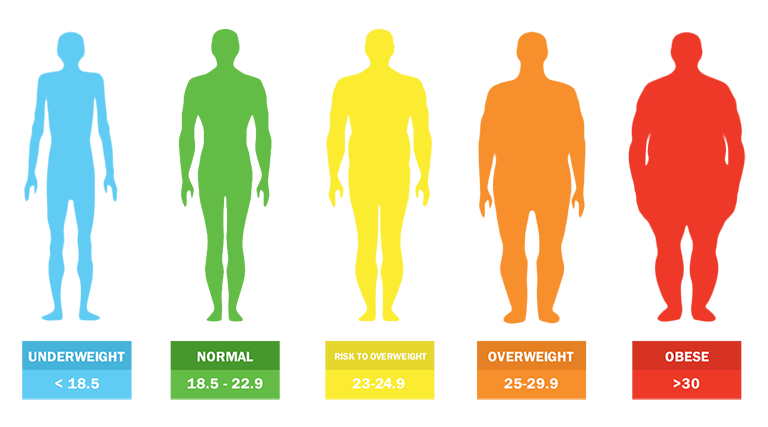## What is the body mass index (BMI)?

### What is the body mass index (BMI)?

The body mass index (BMI) is a measure that uses your height and weight to work out whether your weight is healthy.  BMI is calculated by dividing an adults’s weight in kilograms by their height in meters squared.  For example, A BMI of 25 means 25kg/m2. Read More

### What is the body mass index (BMI)?

The body mass index (BMI) is a measure that uses your height and weight to work out whether your weight is healthy.  BMI is calculated by dividing an adults’s weight in kilograms by their height in meters squared.  For example, A BMI of 25 means 25kg/m2. Read More